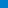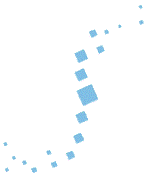Equipe Optimisation CombinatoireSéminaire2022 - 20232021 - 20222019 - 20202018 - 20192017 - 20182016 - 20172015 - 20162014 - 20152013 - 20142012 - 20132011 - 20122010 - 20112009 - 20102008 - 20092007 - 20082006 - 20072005 - 20062004 - 20052003 - 2004EvènementsLiens# Séminaires 2021 - 2022

Ceci est la page web du séminaire de l'équipe Optimisation Combinatoire du laboratoire G-SCOP, à Grenoble.

Sauf mention contraire, le séminaire de Mathématiques Discrètes a lieu le jeudi à 14h30 en Salle C319. Les responsables sont Louis Esperet et András Sebő, n'hésitez pas à les contacter.

Depuis 2020, nous organisons également un séminaire virtuel (conjointement avec Lyon et Clermont-Ferrand), le Séminaire virtuel de théorie des graphes et combinatoire en Rhône-Alpes et Auvergne.

• Jeudi 23 juin 2022 (à 14h30): Felix Klingelhöfer (G-SCOP) : Practical and possibly efficient algorithms for finding maximum stable sets in cycle-plus-triangles graphs

A Cycle-plus-Triangles graph is the union of t triangles and a Hamilton cycle on the 3t vertices and is known to be 3-colorable. There is, however, no known efficient algorithms to find a 3-coloring or even to find a maximum stable set. We present a simple randomized algorithm that outputs a maximum stable set upon termination. We conjecture that for any Cycle-plus-Triangles instance, our algorithm terminates in expected polynomial time. In an (unsuccessful) effort to find hard instances for our algorithm, we discuss structure and properties of Cycle-plus-Triangles instances and methods for generating them.

Joint work with Moritz Mühlenthaler, Alantha Newman and Heiko Röglin.

• Lundi 23 mai 2022 (à 15h): Matthias Englert (University of Warwick, UK) : Improved Approximation Guarantees for Shortest Superstrings

In the Shortest Superstring problem, we are given a set of strings and we are asking for a common superstring, which has the minimum number of characters. The Shortest Superstring problem is NP-hard and several constant-factor approximation algorithms are known for it. Of particular interest is the GREEDY algorithm, which repeatedly merges two strings of maximum overlap until a single string remains. Tarhio and Ukkonen (TCS 1988) conjectured that GREEDY gives a 2-approximation. In a seminal work, Blum, Jiang, Li, Tromp, and Yannakakis (STOC 1991) proved that the superstring computed by GREEDY is a 4-approximation, and this upper bound was improved to 3.5 by Kaplan and Shafrir (IPL 2005). We show that the approximation guarantee of GREEDY is at most (roughly) 3.425. Furthermore, we prove that the Shortest Superstring can be approximated within a factor of 2.475.

Joint work with Nicolaos Matsakis, Pavel Vesely.

• Vendredi 20 mai 2022 (à 14h30): Alantha Newman (G-SCOP) : Correlated Rounding for Correlation Clustering

Given a complete graph G = (V, E) where each edge is labeled + or -, the correlation clustering problem asks to partition V into clusters to minimize the number of +edges between different clusters plus the number of -edges within the same cluster. The approximability of correlation clustering has been actively investigated [BBC04, CGW05, ACN08], culminating in a 2.06-approximation algorithm [CMSY15], based on rounding the standard LP relaxation. Since the integrality gap for this formulation is 2, it has remained a major open question to determine if the approximation factor of 2 can be reached, or even breached. In this talk, we show how to achieve a factor of 2+eps based on O(1/eps^2) rounds of the Sherali-Adams hierarchy. To round this relaxation, we adapt the correlated rounding originally developed for CSPs [BRS11, GS11, RT12]. To go below this approximation ratio, we go beyond the traditional triangle-based analysis by employing a global charging scheme that amortizes the total cost of the rounding across different triangles.

• Jeudi 12 mai 2022 (à 14h30): Valentin Garnero (G-SCOP) : On improving matchings in trees, via bounded-length augmentations

In a graph G, it is well-known that a matching can be turned into a bigger matching by repeatedly applying a so-called augmentation operation. From an initial matching of G, one may naturally ask about the maximum matching that can be reached by performing augmentations.
In a recent work, Nisse, Salch and Weber  considered the influence on this problem of restricting the length k of the augmented paths. As main results, they proved that, for k = 3, reaching a maximum matching from an initial matching can be done in polynomial time for any graph, while the same problem for any k ≥ 5 is NP-hard. In particular, they proved the latter result to remain true for planar bipartite graphs with maximum degree 3 and arbitrarily girth, and left open the complexity of the same problem in the case of trees. As a first step, they nevertheless exhibited a polynomial-time algorithm solving this problem for any path tree.
In this work, we make a further step towards understanding this problem for general trees. We exhibit polynomial-time solving algorithms for a few more tree classes, namely bounded-degree trees when k is a fixed parameter, caterpillars, and trees where the vertices with degree at least 3 are sufficiently far apart. We then consider the influence on this problem of being restricted, for any fixed k ≥ 3, to augment paths of length exactly k only. Contrarily to the initial problem, the problem is already NP-hard for k = 3 in planar bipartite graphs with maximum degree 3 and arbitrarily girth. Finally, we prove that the problem becomes NP-hard for trees when k is part of the input.

Joint work with Julien Bensmail and Nicolas Nisse.

• Jeudi 5 mai 2022 (à 14h30): Thomas Suzan (G-SCOP) : Recoloring Directed Graphs

For a fixed graph H, the H-Recoloring problem asks whether for two given homomorphisms from a graph G to H one can be transformed into the other by changing the color of a single vertex in each step and maintaining a homomorphism to H throughout. In the general case, H-recoloring is PSPACE-complete, but there are some classes of undirected graphs for which a polynomial algorithm is known. For instance, in 2014, M. Wrochna introduced a topological algorithm that solves the H-Recoloring problem in polynomial time when H is square-free. After explaining Wrochna's algorithm, I will show how it can be generalized to solve the H-recoloring problem when H belongs to several classes of directed graphs.

• Jeudi 7 avril 2022 (à 14h30): Nathan Harms (University of Waterloo, Canada) : Adjacency and Distance Sketching for Graph Classes

I will define adjacency and distance sketches for graph classes and give a summary of the research on these objects, including their connections to adjacency & distance labeling schemes and randomized communication complexity. For a graph class C, an adjacency (or distance) sketch assigns random labels to each vertex of a graph G in C, so that adjacency (or distance thresholds) between vertices x and y can be decided with high probability from the labels of x and y, by a decoder who knows the class C but not the specific graph G. Particularly interesting are sketches of constant size, where the size of the random labels does not depend on the size of the graph. Graph classes with constant-size adjacency sketches are equivalent to two-player communication problems with constant-cost randomized protocols, and they are also a subset of the graph classes that admit efficient (deterministic) labeling schemes. I will present some recent results on these constant-size adjacency and distance sketches.

• Jeudi 31 mars 2022 (à 14h30): Moritz Mühlenthaler (G-SCOP) : Matroids, Matchings, and Reconfiguration

We review some recent and not so recent results on the reconfiguration of matroid bases, matchings, and related structures. We identify Matroid Parity as a potential avenue for generalizing known positive results and highlight some of the many interesting special cases of Independent Parity Set Reconfiguration whose complexity is not known. We show that while Independent Parity Set Reconfiguration is PSPACE-complete in general, surprisingly, it admits a polynomial-time algorithm when restricted to non-maximum independent parity sets. We then focus on the reconfiguration variant of the feedback vertex set (FVS) problem, which is, on subcubic graphs, a special case of Linear Matroid Parity. On the positive side, we give a polynomial-time algorithm for FVS Reconfiguration on K33-minor-free graphs and show that the problem is PSPACE-complete on graphs of maximum degree at least four.

• Jeudi 24 mars 2022 (à 14h30): Laurent Feuilloley (LIRIS, Lyon) : Local certification of graph classes

Local certification is a type of graph labeling, coming from distributed computing. For a given graph class X, it allows the vertices of a graph to collectively decide whether the graph they belong to is in X or not, by just looking at the labels of their neighbors. Local certification ends up being a way to study the locality and the constructive characterizations of graph classes.
In this talk, I will introduce the concept of local certification, and survey the recent results of the area, with a focus on H-minor-free classes.

• Jeudi 10 mars 2022 (à 14h30): Marco Caoduro (G-SCOP) : Hitting and Packing Squares

Let S be a family of (not necessarily axis-parallel) squares in the plane. The transversal number of S, denoted by tau(S), is the minimum number of points needed to hit all the squares in S, and the independence number of S, denoted by nu(S), is the maximum number of pairwise disjoint squares in S. Clearly, tau(S) is at least nu(S). We prove that tau(S) ≤ 10 nu(S) and present a family where tau(S) = 3 nu(S). During the talk, we will sketch the proof of the main result and remark how to extend our approach to families of rectangles with bounded aspect ratios.

This is joint work with András Sebő.

• Jeudi 7 octobre 2021 (à 13h30): Imre Bárány (Rényi Institute, Budapest) : Cells in the box and a hyperplane

It is well known that a line can intersect at most 2n-1 cells of the n x n chessboard. What happens in higher dimensions: how many cells of the d-dimensional [0,n]^d box can a hyperplane intersect? We answer this question asymptotically. We also prove the integer analogue of the following fact. If K,L are convex bodies in R^d and K \subset L, then the surface area of K is smaller than that of L.

Joint work with Peter Frankl.

• Jeudi 14 octobre 2021 (à 14h30): Antoine Dailly (G-SCOP) : Equilibrabilité

La théorie de Ramsey consiste en la recherche de structures monochromatiques au sein de très grandes structures colorées arbitrairement. Récemment, des travaux se sont tournés vers des patrons autres que monochromatiques. En particulier, Caro, Hansberg et Montejano ont introduit en 2020 le concept d'équilibrabilité : on cherche à garantir, au sein de toutes les 2-colorations des arêtes de Kn, l'existence de copies équilibrées d'un graphe donné (i.e., avec la moitié des arêtes de chaque couleur). Notons qu'il est nécessaire d'avoir un certain nombre minimum d'arêtes de chaque couleur pour garantir cette existence (sinon, la 2-coloration où toutes les arêtes de Kn sont de la même couleur est toujours un contre-exemple) ; ce nombre définit un paramètre, le nombre d'équilibrage du graphe G, noté bal(n,G). Caro, Hansberg et Montejano ont caractérisé les graphes équilibrables, et étudié l'équilibrabilité et le nombre d'équilibrage des chemins, des arbres et des étoiles. Dans cet exposé, je présenterai des travaux réalisés au cours de mon postdoc au Mexique, en collaboration avec Laura Eslava, Adriana Hansberg et Denae Ventura. Nous avons étudié l'équilibrabilité et le nombre d'équilibrage des cycles, des circulants C(k,l) et de grilles. Nous avons également étudié une variante, le nombre d'équilibrage de liste, qui permet de mesurer à quel point des graphes non-équilibrables sont proches de l'être ou non.